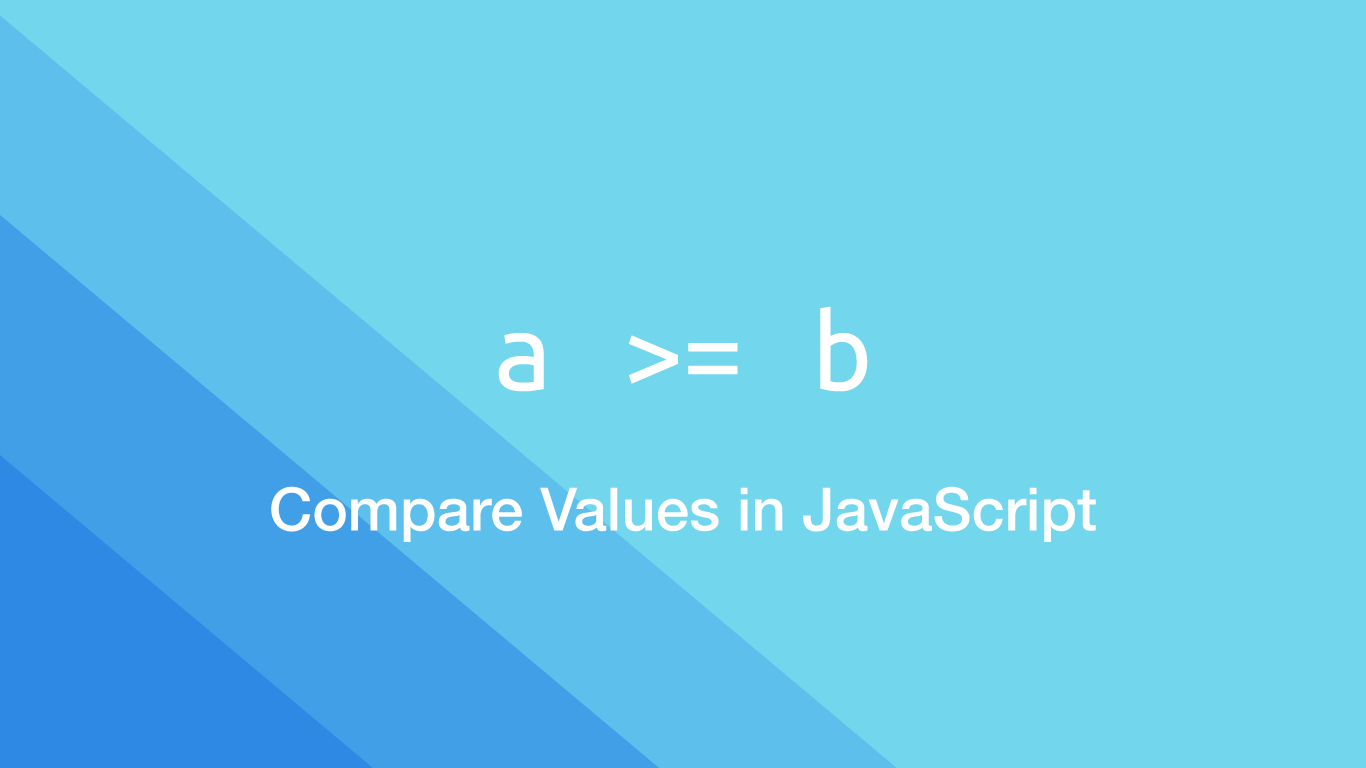# How to Compare Values in JavaScriptComparing values is a fundamental aspect of programming in JavaScript, and programming in general since you will not be able to make a program that does anything too interesting without value comparisons.

In this tutorial we will go over how to compare values in JavaScript, starting from the operators that are available and then how JavaScript behaves with different strings and objects.

## The JavaScript Comparison Operators

Here are the main JavaScript comparison operators that are available.

• `>` - greater than
• `<` - less than
• `==` - equal to; not to be confused with `=` (variable assignment)
• `===` - strict equal to. This takes inputs literally with no conversions
• `>=` - greater than or equal to
• `<=` - less than or equal to
• `!=` - not equal to
• `!==` - strict not equal to

## The Result of a Comparison

All comparison will return `true` or `false`. `true` meaning correct there was a match and `false` for no match.

``````2 = 1
``````
``````false
``````
``````2 != 1
``````
``````true
``````

You can assign the result of a comparison to a variable for use elsewhere in the program.

``````var result = 2 > 1;
``````

## How to Compare Strings in JavaScript

JavaScript handles string comparisons on a character by character basis from the start of each string. Characters are valued from smallest to largest in dictionary order, also known as lexicographical order. `a` is less than `z`,  a number is always less than a letter, and a lowercase letter is always greater than an uppercase one.

``````console.log('a' > 'b');
``````
``````false
``````
``````console.log('a' > 'A');
``````
``````true
``````
``````console.log('a' > '6');
``````
``````true
``````

Since we know that strings are compared with each other one character at a time, it makes sense why the example below returns `true`.

``````console.log('abde' > 'abcd');
``````
``````true
``````

`d` is greater than `c` and as soon as JavaScript sees that it returns `true`. It doesn't matter what comes after d. For the above example to return `false` each letter on the left side of the logic would have to be less than or equal to its counterpart on the right.

## Compare Strings and Number Objects

If a string is compared to a number JavaScript will convert the string into a number. No conversion to a number is necessary.

``````console.log('2' > 1);
``````
``````true
``````

## Strict Equal to

The `==` (equals) operator in JavaScript works when you know exactly what the input will be, in other cases it can produce bugs or unexpected behaviour.

`==` also does not distinguish between `0` and `false`, or an empty string and `false`.

``````console.log(0 == false);
``````
``````true
``````
``````console.log('' == false);
``````
``````true
``````

`===` (strict equals) is a safer way to check if two values are the same. This is because no conversions inside JavaScript itself are made in the process; it simply takes your inputs and checks they match exactly.

``````console.log(0 === false);
``````
``````false
``````
``````console.log('' === false);
``````
``````false
``````

## Strict Not Equal to

You can perform a strict not equal to like this:

``````console.log(0 !== false);
``````
``````true
``````

## Comparing null and undefined

There are two ways to compare `null` and `undefined` depending on whether you need `true` or `false` when comparing them.

``````console.log(null == undefined);
``````
``````true
``````

In the above example JavaScript `==` (equals) converts `null` and undefined to mean the same thing. `===` (strict equals) does not do this:

``````console.log(null === undefined);
``````
``````false
``````

## Conclusion

You now know how to compare strings and other values in JavaScript and what some of their quirks are. JavaScript string comparison is one of the most important to know about since its behaviour is different than one might assume.

#### Related Tutorials### How to Compare Arrays in JavaScript

October 15, 2020### How to use the JavaScript reduce() Method

November 06, 2020### How to use AND, NOT and OR Logical Operators in JavaScript

September 08, 2020### How to Compare Strings in Python

September 20, 2020### How to use the XOR operator in Python

April 07, 2021June 18, 2020